## A

Acceleration Pickup
Transducer which generates output (generally, an electric signal) proportional to the input acceleration.
Acceleration Transducer
Synonym for acceleration pickup.
Accuracy
Coincidence of indicated value with approved standard value or desired value.
Note: The limit of error is used only as a qualitative concept.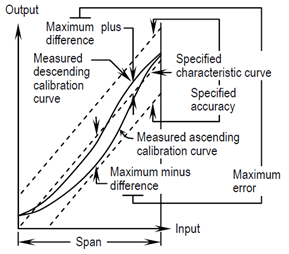Air Purge
Clean air externally supplied to the equipment for the following purposes:
(1) To prevent corrosive gages entering the equipment.
(2) To prevent temperature rise inside the equipment.
(3) To prevent explosive gases entering the equipment for flame proof construction, etc.
Allowable Error
Difference between the preset reference and the allowable limit value.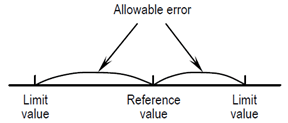Connectable load resistance within the range that the performance is guaranteed.
Amount of Unbalance
Quantitative scale of unbalance (on certain surface) in the rotor, and angular position is not considered.
It is also the product of unbalance mass and mass eccentricity.
Note: Unit of unbalance is g-mm, for example.
Amplitude
Maximal value of sinusoidal quantity.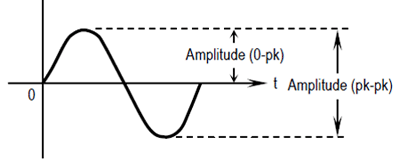Angle of Unbalance
Angle of location where unbalance mass exists with respect to the reference line in the polar coordinates system fixed in the plane perpendicular to the axial center line.
API Standard
Standard defined by the American Petroleum Institute.
Attenuation
(1) Reduction in signal size.
(2) Reduction in vibration amplitude with time.

## B

Balancing
Maintaining vibration or force synchronous with the rotating speed within the specified limit by checking the rotor mass distribution and adjusting the distribution if necessary.
Bode Diagram
A pair of diagrams which show the amplitude ratio and phase shift with respect to frequency for a frequency response.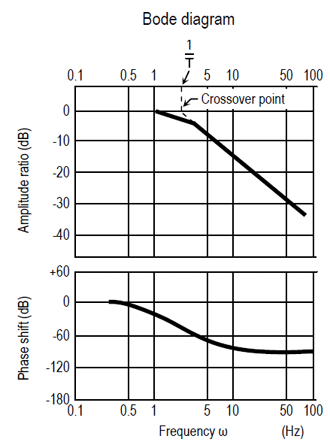## C

Calibration
To determine the equipment output corresponding to a series of known value of the quantity to be measure, received or transmitted.
Note: The following steps are conducted during actual calibration.
(1) To determine each position where a scale line is drawn on a scale plate.
(2) To perform adjustment to match the output with the desired value within an
allowable error stipulated in the specifications.
(3) To determine the error by comparing the equipment output readings with
the standard output values.
(4) Generally, calibration is performed to match independent quantities with
the specifications.
Contact Capacity
Contact capacity expressed by the maximum allowable voltage applicable to the contact part and the maximum allowable current at which the contact part can be opened or closed.
Contact Input
Input of which the signal represents open/close state of an electrical contact.
Contact Output
Output signal generated by an electric contact opening or closing.
Contact Type
Contact type resulting from the difference between circuit configurations of switches, etc.
Example:
Single-pole normally open (SPNO) type.
Signal-pole normally closed (SPNC) type.
Single-pole double-throw (SPDT) type
Double-pole double-throw (DPDT) type.
Crest Factor
Ratio of the peak value to rms value.
Note: The crest factor of a sine wave is 2
Critical Speed
Rotating speed when a rotating system becomes resonant.
Cycle
All states or values in the entire range followed by a cyclic phenomenon of function until returns to the previous status again.

## D

Damped Natural Frequency
Frequency of natural vibration in a linear system having attenuation.
Damper
Device to absorb energy to reduce the shock or amplitude.
Damping Ratio
Ratio of actual damping coefficient to critical damping coefficient in a system having linear Viscous Damping.
Note: The camping ratio is also expressed as a percentage to critical damping.
Delay Time
Time which characterizes the time lag.
Generally, this is expressed as the time until the tangential line drawn at the point with the sharpest gradient on the response curve of the output signal intersects with the time axis just after the step input signal is applied.
Note: This time is also called “effective dead time.”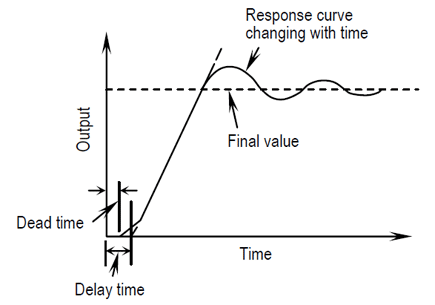Differential Expansion
Measurement of dimensional changes of the gap between the rotor and casing which changes with the difference between the thermal expansion speeds of the rotor and casing.
Digital Signal
Signal having a parameter which gives a set of digitized values displayed numerically.
Displacement Pickup
Transducer which generates an output (generally, an electrical signal) proportional to the input displacement.
Dry Contact Output
Electric contact output which does not feed power to the contact part from the contact activation side.
Dynamic Characteristic
Response characteristics of equipment covering the input changing with time.

## E

Eccentricity P-P
The amount of rotor-shaft deflection produced when the rotor of rotating machinery is at a standstill caused by the dead weight of the rotor itself or at an uneven cooling speed.
Electromagnetic Interference
Unfavorable effect imposed on circuits and parts by an external magnetic field.
Note: Ratio frequency interference (RFI) is a well-known example.
Elliptical Vibration
Vibration whose vibrating points describe an elliptical locus.
Error
Algebraic difference between a measured value and an ideal variable to be measured.
Excitation
External force acting on the system or input.
Explosion Class
Explosion class or ignition temperature of explosive gases existing (or assumed to exist) in the hazardous area where the explosion-proof equipment is installed.
Explosion-proof
When equipment is installed in a hazardous area with explosive gases (inflammable gases or vapor generated from inflammable liquids), protection from explosion or fire caused by the equipment itself.
Explosion-proof Construction
Equipment designed to the explosion-proof.
Note: The following constructions are frequency used for explosion-proof construction of electrical equipment as industrial control equipment:
(1) Flameproof construction
(2) Intrinsically safe construction
(3) Combination of (1) and (2)
External Magnetic Field
Strength of magnetic field existing where the equipment is installed.
(Not the magnetic field generated by the equipment itself.)

## F

Failure Rate
Rate of failure of equipment or system operated until a certain period occurring within the succeeding unit of period.
Field Balancing
Balancing conducted using the bearing of the machine itself to be balanced and the support structure without using a balancing machine.
Flameproof Construction
Explosion-proof construction in which if explosive gases explode in a fully closed enclosure, it is tested and approved by a public agency that the enclosure can withstand the explosion pressure and does not produce flames which may ignite external explosive gases.
Flexible Rotor
Rotor which does not satisfy the definition of rigid rotor.
Flexural Critical Speed
Rotating speed at which one of the main flexural modes of the flexible rotor becomes a maximum. Rotor defection is more important than bearing displacement.
Flexural Principal Mode
Mode a rotor takes through flexural critical speed.
Flexural Vibration
Vibration appears as change in flexure of an elastic body.
FM STANDARD
Abbreviation of Factory Mutual Laboratories Standard defined by an American insurance company.
Form Factor
Ratio of the rms value to the mean value in a half cycle passing through two zero points.
Foundation
Structure which supports a mechanical system.
Note: This may be fixed in space or may give excitation to the supported mechanical system by its motion.
Free Vibration
Vibration without excitation.
Note: In free vibration, a system vibrates at its natural frequency.
Frequency
Number of cycles/second.
Frequency Analysis
Spectrum analysis of vibration phenomenon.
Frequency Response (Characteristic)
Time response of the output signal when the input signal changes sinusoidally.
Generally, it is expressed using a Bode diagram.
Fundamental Natural Mode of Vibration
Vibration mode having the minimum natural frequency.

## H

Harmonic
Sinusoidal quantity with a frequency equal to an integer times the fundamental frequency.
Note: A sinusoidal quantity with a frequency equal to an integer of 2 or more times the fundamental frequency is called a higher harmonic.
High-Speed Balancing
Balancing performed at a rotating speed under which the rotor cannot be assumed to be a rigid body.
Hysteresis
Dependency of output value occurring from the past record and direction of changes with respect to changes of a given input.
Note:
(1) Generally, hysteresis is obtained by subtracting the dead band value from the
maximum measurement interval occurring between the forward and backward
travels of the measured-quantity indication (or output) after the transient state
ahs settled.(unless otherwise noted, the indication or output is changed over
the entire range.)
(2) If an extremely small input is reversed a few times, output is also expected to
be reversed a few times. According to this method, the dead band is distinguished
from the hysteresis.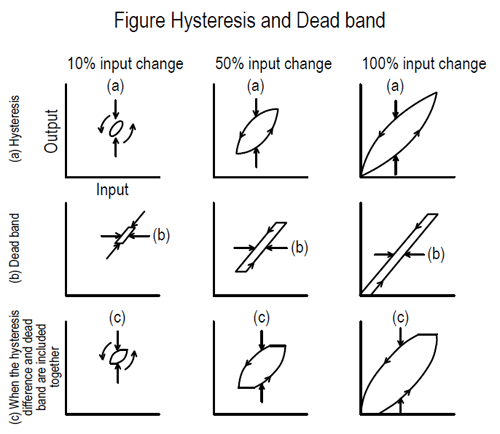## I

Input Bias
Bias added to the input signal.
Input Impedance
Impedance included in a circuit of a device when its inside is looked from the input terminal.
Input/Output Isolation
Synonym for isolation.
Input Resistance
Resistance component in a circuit when the equipment is viewed from the input terminal side of the equipment.
Insulation Resistance
Electric resistance between two conductors insulated by an insulator.
Example: More than 20 MΩ at 500 VDC (between each power terminal and the grounding terminal)
Interchangeability
Capable of being mutually interchanged.
For products, there is dimensional or characteristic interchangeability.
Internal Resistance
Resistance component in a circuit when the equipment is viewed from the input or output terminal side of the equipment.
Intrinsically Safe Construction
Explosion proof construction that the electric spark during normal operation or in a failure state, or heated part will not ignite the surrounding explosive gas, which has been proven by an official body’s evaluation or equivalent.
Isolation
No common line should exist between input and output signals of equipment.
Isolation between input and output signals of equipment.

## L

Linearity
Linearity obtained when the maximum deviation between a calibration curve and the specified straight line is approximated to become a minimum.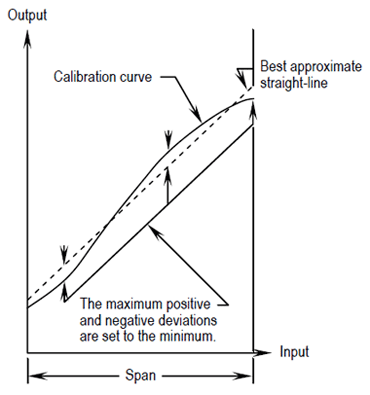Linear Range
Measurement range that satisfies the specifications of a transducer, such as sensitivity, scale factor, linearity, etc.
Linear System
System whose response is proportional to the size of excitation.
Note: According to this definition, the dynamic characteristic of each element in the system is expressed by a series of linear differential equations and the principle of superposition can be applied to this system.
Linear Transducer
Transducer whose output is proportional to input within the specified frequency range.
Linear Viscous Damping Coefficient / Viscous Damping Coefficient
Ratio of damping force to speed.
Linear Viscous Damping / Viscous Damping
Energy consumed when an element or a part of an element in a vibration system receives a resistance for proportional to the element speed in the opposite direction.
Lissajous Figure
Steady diagram draw when two simple harmonic motions whose frequency rates are expressed by a simple integer ratio are initiated in mutual directions at right angles.
Note: The Lissajous figure varies with the difference of phase angles of simple harmonic motions.
Part connected to the output side of equipment and consuming or absorbing output energy.
Low-speed Balancing
Balancing performed at a rotating speed at which the rotor is assumed to be a rigid body (generally, the speed slower than the operating speed).

## M

Mass Eccentricity
Distance from the shaft center line to the rotor center of gravity.
Maximum load which can be connected to the output side of the equipment.
Measuring Period
Time interval between the present and nest measurements when measuring at fixed intervals.
Measuring Range
Range of the maximum and minimum measured variables.
Measuring Span
Difference between measurement result is obtained from the start of measurement.
Measuring Time
Time required from measurement start to acquirement of measurement result.
Modal Analysis
(1) To conduct response analysis in each mode and to obtain the response waveform
of the entire system by adding response waveform.
(2) To obtain the maximum response value in each mode and to obtain the estimated
maximum response value of the entire system by an appropriate method of addition.
Modal Balancing
Balancing operation to correct the flexible-rotor vibration amplitude to within the specified limit for each mode.
MTBF (Mean Time Between Failures)
Mean time between consecutive failures occurring in the specified period, and condition within the service life of each equipment.
MTTR (Mean Time to Repair)
Mean time required for repair work.

## N

Natural Mode of Vibration
vibration mode in a freely vibrating system.
Notes:
(1) If there is no attenuation in the system, the natural mode is the same as the normal mode.
(2) The natural mode of vibration exists corresponding to each degree of freedom in the system.
NC (Normally Closed)
Contact which opens when the contact is activated or at power-ON.
Note: Also called “b” contact.
NO (Normally Open)
Contact which closes when the contact is activated or at power-ON.
Note: Also called “a” contact.
Nyquist Diagram
Technique of evaluating the safety in a control system conceived by H. Nyquist.
Diagram which shows the shaft vibration vector of rotating machinery on polar coordinates with the number of revolutions as a parameter.

## O

Oil-Whip
Self-excited journal whirling caused by the properties of the oil film on a fully lubricated journal bearing.
Open Collector (Contact)
Transistor contact without collector load resistance on the contact activation signal.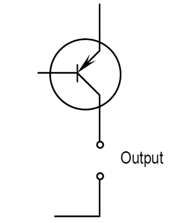Output Bias
Bias added to the output signal.
Output Contact Capacity
Maximum current which can be supplied to the load while maintaining the specified performance of the voltage output equipment.
Output Current Capacity
On equipment with current output, the maximum amount of current that can be supplied to a load within a range that a certain level of performance can be maintained.
Output Impedance
Impedance included in the internal circuit of equipment when viewed from the output terminal side.
Output Resistance
Resistance component included in the internal circuit of the equipment when viewed from the output terminal side.
Outside Contact Capacity
Outside contact capacity when equipment with a built-in power supply supplies power to an outside contact and the equipment is operated by that outside contact.

## P

Peak-to-Peak Value
The maximum algebraic difference between extreme values of vibrating quantity in the given section.
Peak Value
Maximal value in the given section.
Note: Generally, the peak value of a vibrating quantity is defined as the maximum variation from the mean value in the given section. The positive maximum variation is called the positive peak value, and the negative maximum variation is called the negative peak value.
Period
Inverse number of frequency.
Phase Angle/Phase
Lead angle of sinusoidal quantity measured from an independent variable set as a reference.
Phase Difference
Difference between phase angles existing in two periods of equal frequency.
For a sinusoidal quantity, the difference between phase angles measured from the same reference.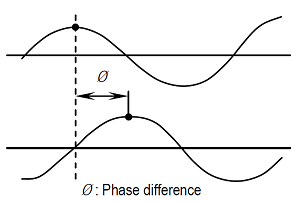Phase Marker
Rotor-shaft phase reference signal used for comparing the vibration phase of rotating machinery.
Pulse Interval
Time interval from one pulse to the next pulse.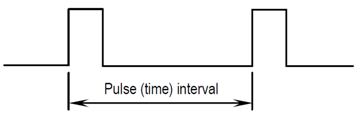Pulse Voltage
(1) Broadly: pulse voltage defined by the specified method.
(2) Detailed: Voltage obtained by subtracting the pulse base voltage
(voltage below the base line) from the pulse top voltage defined by the common
method.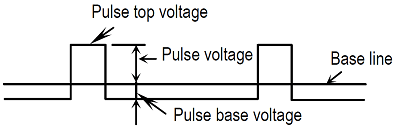Pulse Width
Duration of pulse. Unless otherwise specified, the time from the pulse rise mesial point to the pulse fall mesial point.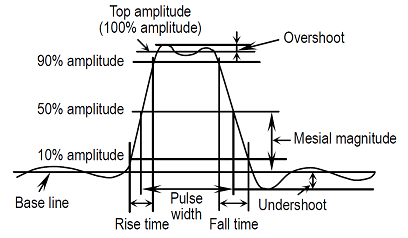## Q

Q
Degree of sharpness of resonance in a vibration system. It is defined as 2 times the ratio of energy/cycle externally applied in order to maintain a constant amplitude to energy (in a mechanical oscillating system, the sum of kinetic energy and potential energy) in the vibration system at the resonance frequency.

## R

Random Failure
Failure accidentally occurring before the wear-out failure period following the period of initial failures.
Range
Range between the maximum and minimum values of input or output variables.
Reliability
Capability for displaying a required function of the equipment in the specified period and condition.
Reproducibility
The maximum output difference obtained when the same input is repeatedly measured by approaching it from both directions under the same operating conditions after a fixed time.
Resistance Against Vibration
Strength against externally-applied vibration.
Note: Since the strength varies with the vibration frequency and amplitude, resistance against vibration should be expressed together with the allowable limits of frequency and amplitude or acceleration.
Load consisting only of electrical resistance components.
Resolution
Minimum interval between two mutually distinguishable, adjacent values.
Resonance
Status or phenomenon occurring in a forced vibration system when the response lessens by slight changes in the excitation frequency in any directions.
Resonance Frequency
Frequency at which resonance occurs.
Response Time
Time required until the output signal arrives at the specified value of the final value by applying a step input signal.
Generally, the time to arrive at 90%, 95% or the final value is used, but the time to arrive at 63.2% of the final value (time constant) is used unless otherwise specified.
Note: When response time only is used, this means step response time.
Rigid Rotor
Rotor whose unbalance does not exceed the allowable error (with respect to the shaft center line) by rotor deformation, even when rotated at a speed of less than the maximum speed under the support condition close to the operating condition while balanced between two freely selected planes.
RMS Value
Root Mean Square Value.
Note: Also called effective value.
Run Out
When an eddy-current non-contact displacement sensor detects vibration and eccentricity of rotating machinery this is a measurement error caused by the rotor surface status. It is classified into mechanical runout and electrical runout.
Their causes are as follows:
For mechanical runout;
Rotor bending, uneven surface
For electrical runout;
Residual magnetic field, non-uniform material, etc.

## S

Sampling
To capture instantaneous input values at a preset time (for example, at fixed intervals).
Sampling Period
Time intervals of observation in a periodic sampling control system.
Sampling Time
Time to sense the input during sampling.
Scale Factor
Output changes per specified length in the static eddy-current displacement sensor characteristic.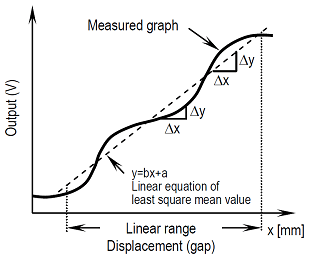Scale Factor Error
Difference between a specified sensitivity (s) and the measured scale factor expressed on percentage.
Scale Factor Error = {(SCF-s)/s} × 100[%]
Secular Change
Characteristic changes occurring in the equipment or its elements with time.
Seismic Pickup
Transducer which consists of a seismic system and generates an output signal as a result of relative motion between two elements in the seismic system.
Seismic System
System having one mass element installed via a base frame and one or more spring elements attached to the frame. Generally, damping elements are also included in this system.
Self-excited Vibration
Vibration occurring after non-vibrating energy is converted to vibration excitation in the system.
Sensitivity
Ratio of change in quantity to be indicated to change in quantity to be measured of the equipment.
Sensitivity Error
Difference between a measured value (b) and a specified sensitivity (s) expressed on percentage.
Sensitivity Error = {(b – s)/s} × 100 [%]
SN Ratio (S/N)
(1) Ratio of noise amplitude to signal amplitude.
Note: For a sinewave signal, the peak value or rms value is used. For a non-sinewave
signal, the peak value is used.
(2) Ratio of signal power to noise power.
Note: For impulse noise, the ratio of signal peak value is used.
Span
Difference between high and low range limits.
To adjust the span of equipment to the specified value by an electrical or mechanical operation.
Span Setting
To set the span of equipment to the specified value or position by an electrical or mechanical operation.
Spectrum
Description of a certain quantity as a function of frequency or wave-length.
Static Characteristics
Response characteristics of the equipment with respect to the measured variable which do not change with time.
Step Response
Time response occurring when one of the input variables change stepwise.
Sub Harmonic
Sinusoidal quantity having cycles equal to an integer of 2 or more times the basic excitation cycle.
Sweep
Process in which a controlled variable (generally, frequency) of a vibration generator continuously passes through a certain range.
Sweep Rate
Rate of change of a controlled variable (generally, frequency) of a vibration generator.
Example: Sweep speed = df/dt
Where, f = frequency, t = time

## T

Temperature Drift
Drift caused by changes in the ambient temperature.
TIIS Standard
Standard defined by Technology Institution of Industrial Safety (TIIS), Japan.
Time Constant
Time required to arrive at 63.2% of the final output value in a linear 1st-order lag system by applying a step input.
(1) Time constant with time response expressed by A(1-e-t/T).
(2) In a higher-order lag system, each 1st order lag element in a process has a time constant.
The corner frequency is expressed by ω = 1/T in the Bode diagram.
Time Response
Time changes in the output variable caused by changes in the input variable.
Torsional Vibration
Vibration which occurs as torsional changes of a rigid body.
Transfer Function
Arithmetic relationship between the output and input in a system.
Note: Generally, the transfer function is given as a function of the frequency and is a function of a complex variable.
Transient Response
Time required until an output (signal) returns to steady state when an input (signal) changes to another steady state.
Transmission Distance
Length of an electrical conductor that can transmit signals accurately at a required transmission speed.
Note: Transmission distance is decided based on the conditions including impedances of electrical conductor, device specific input/output characteristics, external noises, property of the measurement system, etc.
Transverse Sensitivity
Sensitivity of excitation in any direction at right angles to the sensing shaft.
Note: This is a function of the excitation direction.
Trigger Voltage
Pulse voltage to trigger rapid changes in status or output of an electronic circuit.

## U

Unbalance
Rotor status of producing a vibration forcer or motion on the shaft as a result of centrifugal force.
Note: Sometimes used as a synonym for the size of unbalance or the vector of unbalance.
Unbalance Mass
Mass considered to exist in the specific radius position and whose product with centripetal acceleration becomes equal to the unbalance force.
Unbalance Tolerance
Size of rigid-rotor shaft unbalance defined as the tolerable maximum value within a plane at right angles to the shaft (corrected or measured surface).
Undamped Natural Frequency
Frequency of free vibration generated only by system inertia and elastic force.
Note: Simply called natural frequency, if this does not cause confusion.

## V

Velocity Pickup
Sensor which generates an output (generally, electrical output) proportional to the input speed.
Velocity Transducer
Measures velocity in millimeter per second. Usually IEPE (Integrated electronics Piezoelectric) type or moving coil type.
Vibration Proof
Synonym for resistance against vibration.
Vibration Vector
Vibration signal expressed with a phase angle with respect to the reference (e.g. keyway) on a rotor and also with vibration amplitude.
Vibrometer
Measuring instrument capable of indicating the size of vibration expressed by the peak value, rms value, etc. on the scale.

## W

Waterproof Construction
Construction of equipment or system installed outdoors without a cover which protects it from the effects of wind and rain.
There are the following types of water-proofing:
Water-proof types: Drip-proof, Rain-proof, Splash-proof, Water injection-proof, Water-proof, Water immersion-proof, Water dipping-proof and Humidity-proof.
Note: For the definition of each water-proof term, see JIS C0920.
Wave Length
Distance a wave travels in one cycle.
Whirling
Turning motion of the shaft center line.
Withstand Voltage (Electric Strength)
Voltage which the insulation of electrical equipment can withstand over a fixed period.
Example: 1 minute at a withstand voltage of 1000VAC (between each power terminal and the grounding terminal)